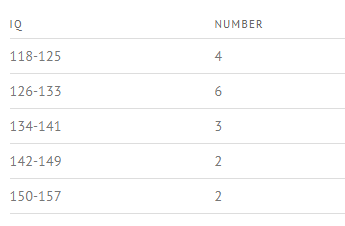# Class Width: Simple Definition

Share onA frequency distribution table showing a class width of 7 for IQ scores (e.g. 125-118 = 7)

Class width refers to the difference between the upper and lower boundaries of any class (category). Depending on the author, it’s also sometimes used more specifically to mean:

• The difference between the upper limits of two consecutive (neighboring) classes, or
• The difference between the lower limits of two consecutive classes.

Note that these are different than the difference between the upper and lower limits of a class.

## Calculating Class Width in a Frequency Distribution Table

Watch the video to find out how to calculate class width:

In a frequency distribution table, classes must all be the same width. This makes it relatively easy to calculate the class width, as you’re only dealing with a single width (as opposed to varying ones). To find the width:

1. Calculate the range of the entire data set by subtracting the lowest point from the highest,
2. Divide it by the number of classes.
3. Round this number up (usually, to the nearest whole number).

## Example of Calculating Class Width

Suppose you are analyzing data from a final exam given at the end of a statistics course. The number of classes you divide them into is somewhat arbitrary, but there are a few things to keep in mind:

• Make few enough categories so that you have more than one item in each category.
• Choose a number that is easy to manipulate; usually, something between five and twenty is a good idea. For example, if you are analyzing a relatively small class of 25 students, you might decide to create a frequency table with five classes.

Example: Find a reasonable class with for the following set of student scores:
52, 82, 86, 83, 56, 98, 71, 91, 75, 88, 69, 78, 64, 74, 81, 83, 77, 90, 85, 64, 79, 71, 64, and 83.

1. Find the range by subtracting the lowest point from the highest: the difference between the highest and lowest score: 98 – 52 = 46.
2. Divide it by the number of classes: 46/5, = 9.2.
3. Round this number up: 9.2≅ 10.

## References

Gleaton, James U. Lecture Handout: Organizing and Summarizing Data. Retrieved from http://www.unf.edu/~jgleaton/LectureTransCh2.doc on August 27, 2018.
Gonick, L. (1993). The Cartoon Guide to Statistics. HarperPerennial.
Jones, James. Statistics: Frequency Distributions & Graphs. Retrieved from https://people.richland.edu/james/lecture/m170/ch02-def.html on August 27, 2018.
Levine, D. (2014). Even You Can Learn Statistics and Analytics: An Easy to Understand Guide to Statistics and Analytics 3rd Edition. Pearson FT Press

---------------------------------------------------------------------------Need help with a homework or test question? With Chegg Study, you can get step-by-step solutions to your questions from an expert in the field. Your first 30 minutes with a Chegg tutor is free!